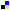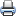# Fiber-Optic TechnologiesSaveDiggDel.icio.usPrint

### Chapter Description

Vivek Alwayn discusses in this chapter the increasing demand of optical-fiber and its wide spread applications ranging from global networks to desktop computers.

## The Physics Behind Fiber Optics

A fiber-optic cable is composed of two concentric layers, called the core and the cladding, as illustrated in Figure 3-1. The core and cladding have different refractive indices, with the core having a refractive index of n1, and the cladding having a refractive index of n2. The index of refraction is a way of measuring the speed of light in a material. Light travels fastest in a vacuum. The actual speed of light in a vacuum is 300,000 kilometers per second, or 186,000 miles per second.Figure 3-1 Cross Section of a Fiber-Optic Cable

The index of refraction is calculated by dividing the speed of light in a vacuum by the speed of light in another medium, as shown in the following formula:

```Refractive index of the medium = [Speed of light in a vacuum/Speed of light
in the medium]```

The refractive index of the core, n1, is always greater than the index of the cladding, n2. Light is guided through the core, and the fiber acts as an optical waveguide.

Figure 3-2 shows the propagation of light down the fiber-optic cable using the principle of total internal reflection. As illustrated, a light ray is injected into the fiber-optic cable on the left. If the light ray is injected and strikes the core-to-cladding interface at an angle greater than the critical angle with respect to the normal axis, it is reflected back into the core. Because the angle of incidence is always equal to the angle of reflection, the reflected light continues to be reflected. The light ray then continues bouncing down the length of the fiber-optic cable. If the angle of incidence at the core-to-cladding interface is less than the critical angle, both reflection and refraction take place. Because of refraction at each incidence on the interface, the light beam attenuates and dies off over a certain distance.Figure 3-2 Total Internal Reflection

The critical angle is fixed by the indices of refraction of the core and cladding and is computed using the following formula:

`qc = cos–1 (n2/n1)`

The critical angle can be measured from the normal or cylindrical axis of the core. If n1 = 1.557 and n2 = 1.343, for example, the critical angle is 30.39 degrees.

Figure 3-2 shows a light ray entering the core from the outside air to the left of the cable. Light must enter the core from the air at an angle less than an entity known as the acceptance angle (a):

`qa = sin–1 [(n1/n0) sin(qc)]`

In the formula, n0 is the refractive index of air and is equal to one. This angle is measured from the cylindrical axis of the core. In the preceding example, the acceptance angle is 51.96 degrees.

The optical fiber also has a numerical aperture (NA). The NA is given by the following formula:

`NA = Sin qa = ?(n12 – n22) `

From a three-dimensional perspective, to ensure that the signals reflect and travel correctly through the core, the light must enter the core through an acceptance cone derived by rotating the acceptance angle about the cylindrical fiber axis. As illustrated in Figure 3-3, the size of the acceptance cone is a function of the refractive index difference between the core and the cladding. There is a maximum angle from the fiber axis at which light can enter the fiber so that it will propagate, or travel, in the core of the fiber. The sine of this maximum angle is the NA of the fiber. The NA in the preceding example is 0.787. Fiber with a larger NA requires less precision to splice and work with than fiber with a smaller NA. Single-mode fiber has a smaller NA than MMF.Figure 3-3 Acceptance Cone

### Performance Considerations

The amount of light that can be coupled into the core through the external acceptance angle is directly proportional to the efficiency of the fiber-optic cable. The greater the amount of light that can be coupled into the core, the lower the bit error rate (BER), because more light reaches the receiver. The attenuation a light ray experiences in propagating down the core is inversely proportional to the efficiency of the optical cable because the lower the attenuation in propagating down the core, the lower the BER. This is because more light reaches the receiver. Also, the less chromatic dispersion realized in propagating down the core, the faster the signaling rate and the higher the end-to-end data rate from source to destination. The major factors that affect performance considerations described in this paragraph are the size of the fiber, the composition of the fiber, and the mode of propagation.

### Optical-Power Measurement

The power level in optical communications is of too wide a range to express on a linear scale. A logarithmic scale known as decibel (dB) is used to express power in optical communications.

The wide range of power values makes decibel a convenient unit to express the power levels that are associated with an optical system. The gain of an amplifier or attenuation in fiber is expressed in decibels. The decibel does not give a magnitude of power, but it is a ratio of the output power to the input power.

`Loss or gain = 10log10(POUTPUT/PINPUT)`

The decibel milliwatt (dBm) is the power level related to 1 milliwatt (mW). Transmitter power and receiver dynamic ranges are measured in dBm. A 1-mW signal has a level of 0 dBm.

Signals weaker than 1 mW have negative dBm values, whereas signals stronger than 1 mW have positive dBm values.

`dBm = 10log10(Power(mW)/1(mW))`
4. Optical-Cable Construction | Next Section Previous Section

There are currently no related articles. Please check back later.